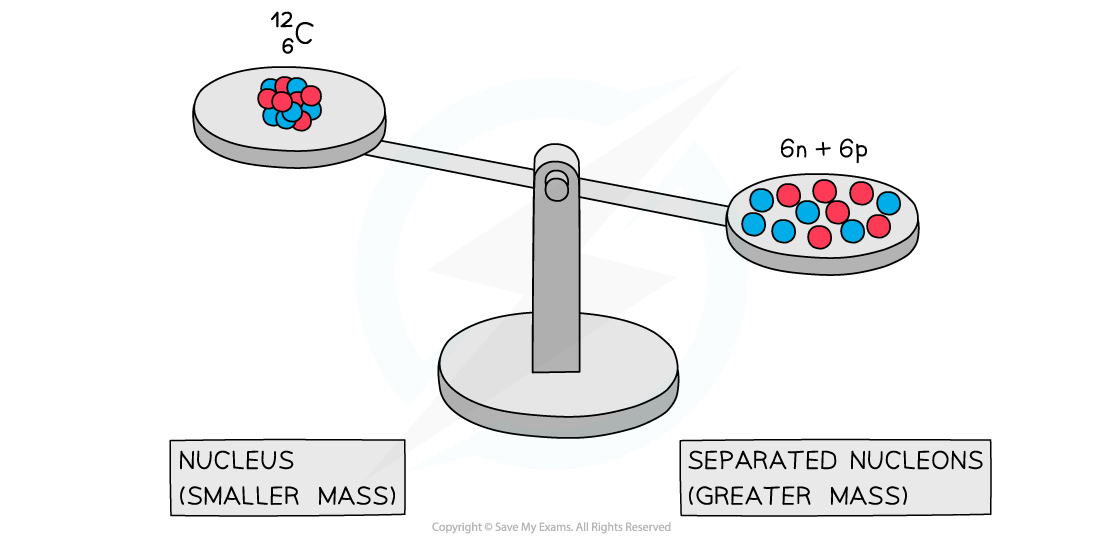# IB DP Physics: HL复习笔记7.2.2 Mass Defect & Nuclear Binding Energy

### Mass Defect & Nuclear Binding Energy

#### Energy & Mass Equivalence

• Einstein showed in his Theory of Relativity that matter can be considered a form of energy and hence, he proposed:
• Mass can be converted into energy
• Energy can be converted into mass
• This is known as mass-energy equivalence, and can be summarised by the equation:

E = mc2

• Where:
• E = energy (J)
• m = mass (kg)
• c = the speed of light (m s-1)
• Some examples of mass-energy equivalence are:
• The fusion of hydrogen into helium in the centre of the sun
• The fission of uranium in nuclear power plants
• Nuclear weapons
• High-energy particle collisions in particle accelerators

#### Mass Defect & Binding Energy

• Experiments into nuclear structure have found that the total mass of a nucleus is less than the sum of the masses of its constituent nucleons
• This difference in mass is known as the mass defect
• Mass defect is defined as:

The difference between an atom's mass and the sum of the masses of its protons and neutrons

• The mass defect Δm of a nucleus can be calculated using:

Δm = Zmp + (A – Z)mn – mtotal

• Where:
• Z = proton number
• A = nucleon number
• mp = mass of a proton (kg)
• mn = mass of a neutron (kg)
• mtotal = measured mass of the nucleus (kg)A system of separated nucleons has a greater mass than a system of bound nucleons

• Due to the equivalence of mass and energy, this decrease in mass implies that energy is released in the process
• Since nuclei are made up of neutrons and protons, there are forces of repulsion between the positive protons
• Therefore, it takes energy, ie. the binding energy, to hold nucleons together as a nucleus
• Binding energy is defined as:

The energy released when a nucleus forms from constituent nucleons

OR

The (minimum) energy needed to break a nucleus up into its constituent nucleons (protons and neutrons)

• Energy and mass are proportional, so, the total energy of a nucleus is less than the sum of the energies of its constituent nucleons
• The formation of a nucleus from a system of isolated protons and neutrons is therefore an exothermic reaction - meaning that it releases energy
• This can be calculated using the equation:

E = Δmc2

#### Exam Tip

Avoid describing the binding energy as the energy stored in the nucleus – this is not correct – it is energy that must be put into the nucleus to pull it apart.

### Calculating Mass Defect & Nuclear Binding Energy

• Binding energy can often be described per nucleon
• This is simply found by dividing the relevant binding energy by the number of nucleons in the nucleus

#### Worked Example

The binding energy per nucleon is 7.98 MeV for an atom of Oxygen-16 (16O).

Determine an approximate value for the energy required, in MeV, to completely separate the nucleons of this atom.

Step 1: List the known quantities

• Binding energy per nucleon, E = 7.98 MeV

Step 2: State the number of nucleons

• The number of nucleons is 8 protons and 8 neutrons, therefore 16 nucleons in total

Step 3: Find the total binding energy

• The binding energy for oxygen-16 is:

7.98 × 16 = 127.7 MeV

Step 4: State the final answer

• The approximate total energy needed to completely separate this nucleus is 127.7 MeV

#### Worked Example

Calculate the binding energy per nucleon, in MeV, for the radioactive isotope potassium-40 (19K).

You may use the following data:

• Nuclear mass of potassium-40 = 39.953 548 u
• Mass of one neutron = 1.008 665 u
• Mass of one proton = 1.007 276 u

Step 1: Identify the number of protons and neutrons in potassium-40

• Proton number, Z = 19
• Neutron number, N = 40 – 19 = 21

Step 2: Calculate the mass defect, Δm

• Proton mass, mp= 1.007 276 u
• Neutron mass, mn= 1.008 665 u
• Mass of potassium-40, mtotal= 39.953 548 u

Δm = Zmp + Nmn – mtotal

Δm = (19 × 1.007276) + (21 × 1.008665) – 39.953 548

Δm = 0.36666 u

Step 3: Convert mass units from u to kg

• 1 u = 1.661 × 10–27 kg

Δm = 0.36666 × (1.661 × 10–27) = 6.090 × 10–28 kg

Step 4: Write down the equation for mass-energy equivalence

E = Δmc2

• Where c = 3.0 × 108 m s–1

Step 5: Calculate the binding energy, E

E = 6.090 × 10–28 × (3.0 × 108)2 = 5.5 × 10–11 J

Step 6: Determine the binding energy per nucleon and convert J to MeV

• Take the binding energy and divide it by the number of nucleons
• 1 MeV = 1.6 × 10–13 J